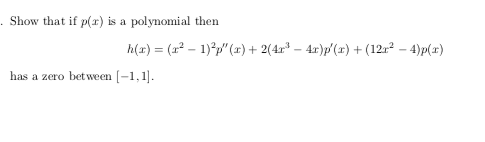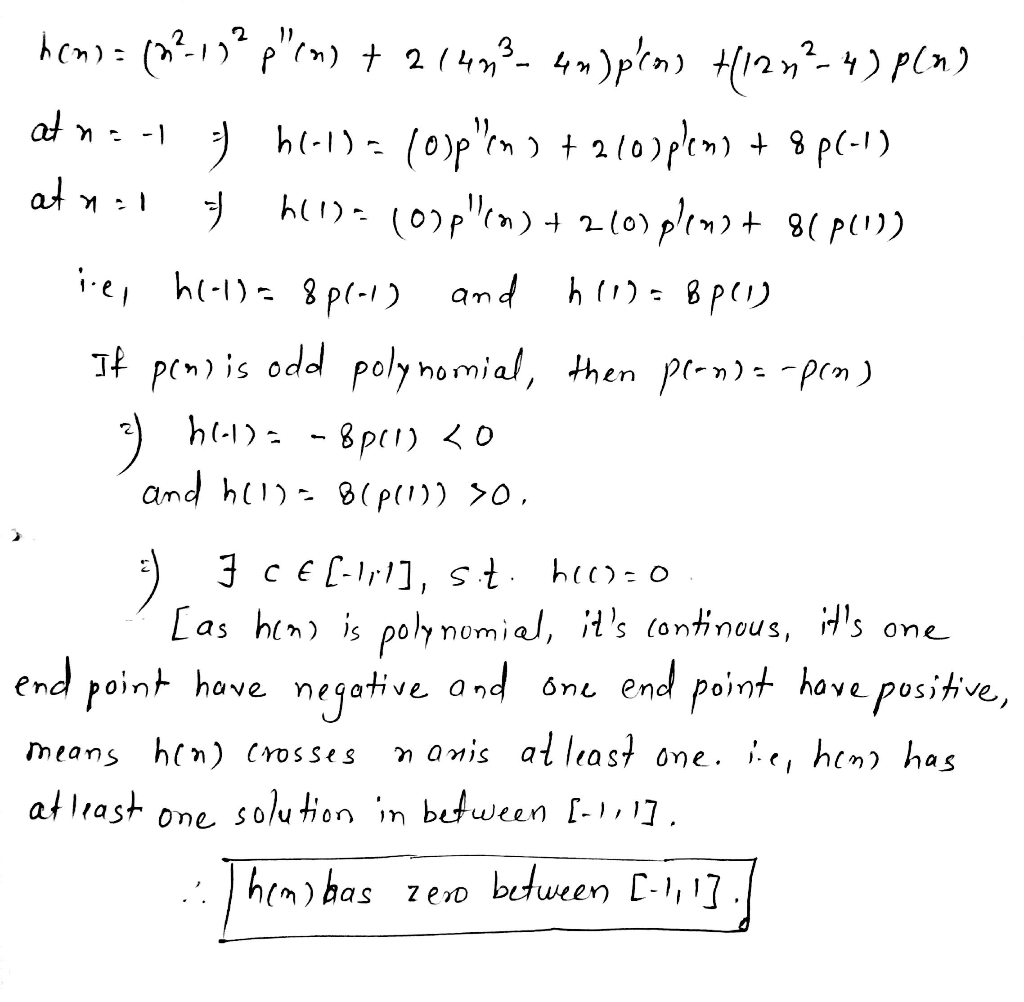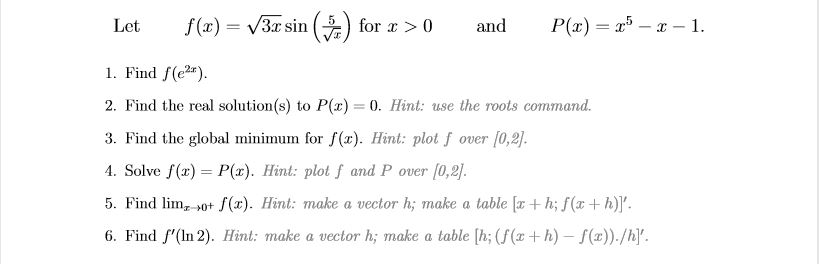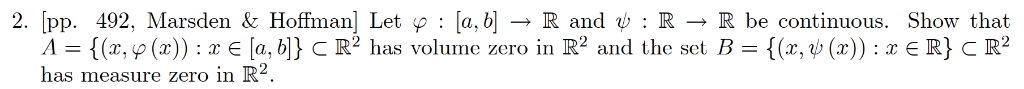# Show that if p(r) is a polynomial then h(x) (2-1)2")2(44)(x)122 -4)px) has a zero bet ween [-1,1Show that if p(r) is a polynomial then h(x) (2-1)2")2(44)(x)122 -4)px) has a zero bet ween [-1,1##### Add Answer of: Show that if p(r) is a polynomial then h(x) (2-1)2")2(44)(x)122 -4)px) has a zero bet ween [-1,1
Similar Homework Help Questions
• ### The polynomial 2x^3+px^2-x+6 has a remainder of zero when divided by x-2

The polynomial 2x^3+px^2-x+6 has a remainder of zero when divided by x-2. Calculate p.

• ### A polynomial of degree 4 has a zero of multiplicity 2 at x=1, and a zero at x=1 2...continues

A polynomial of degree 4 has a zero of multiplicity 2 at x=1, and a zero at x=1 2i.A) Write a polynomial that satisfies the condition (write in expanded form)

• ### The expression px^4+qx^3+3x^2+2x+3 has r(x)=(x+1) when divided by x^3-3x+2

The expression px^4+qx^3+3x^2+2x+3 has r(x)=(x+1) when divided by x^3-3x+2.find the values of p and q.

• ### Use the Intermediate Value Theorem to show that the polynomial function has a zero in the given interval

1. Use the Intermediate Value Theorem to show that the polynomial function has a zero in the given interval. f(x) = 13x^4 - 5x^2 +7x -1; [3,0] Enter the value of (-3). 2. Use the Intermediate Value Theorem to show that the polynomial function has a zero in the given interval. f(x) = 2x^3 + 8x^2 - 7x + 9; [-9, -4] Find the value of (-9)

• ### Let f(z) = V3z sin(#) and P(z) =r-x-1. 1. Find f(e) 2. Find the real solution(s) to Px) 0. Hint: ...This is a MATLAB question so please answer them with MATLAB steps. Let f(z) = V3z sin(#) and P(z) =r-x-1. 1. Find f(e) 2. Find the real solution(s) to Px) 0. Hint: use the roots command. 3. Find the global minimum for f(x). Hint: plot f over [0,2] 4. Solve f()P. Hint: plot f and P over [0,21. 5. Find lim,→0+ f(x). Hint: make a vector hi make a table [x + h; f(x + h)]". 6. Find '(In 2)....

• ### Consider polynomial interpolation of the function f(x)=1/(1+25x^2) on the interval [-1,1] by (1) ...

Consider polynomial interpolation of the function f(x)=1/(1+25x^2) on the interval [-1,1] by (1) an interpolating polynomial determined by m equidistant interpolation points, (2) an interpolating polynomial determined by interpolation at the m zeros of the Chebyshev polynomial T_m(x), and (3) by interpolating by cubic splines instead of by a polynomial. Estimate the approximation error by evaluation max_i |f(z_i)-p(z_i)| for many points z_i on [-1,1]. For instance, you could use 10m points z_i. The cubic spline interpolant can be determined in...

• ### u(x,y)= x+3y,INCOME=12;px =1,py =2;p′x =1,p′y =4 initial prices px,py and final prices p′x,p′y. For THE problem,...

u(x,y)= x+3y,INCOME=12;px =1,py =2;p′x =1,p′y =4 initial prices px,py and final prices p′x,p′y. For THE problem, you are to find: (a) the optimal choice at the initial prices (b) the optimal choice at the final prices (c) the change = optimal choice at final prices - optimal choice at initial prices (d) the income effect and the substitution effect

• ### A polynomial function f(x) has a zero of 3 with multiplicity 2

A polynomial function f(x) has a zero of 3 with multiplicity 2. (1)since the zero is 3, the graph crosses the y-axis at 3? (2) since the zero is 3, the graph goes up to the right? (3) since the multiplicity is 2, the graph crosses the x-axis? (4) since the multiplicity is 2, the graph touches but does not cross the x-axis? Please help me with this!!!

• ### 2. Let f R R and g R-R be functions that are continuous on1,1 and differentiable on (1,1). Suppose that f(-1-f(1) and 9(-1). Show that there exists c e (1,1) such that 2. Let f R R and g R-R...2. Let f R R and g R-R be functions that are continuous on1,1 and differentiable on (1,1). Suppose that f(-1-f(1) and 9(-1). Show that there exists c e (1,1) such that 2. Let f R R and g R-R be functions that are continuous on1,1 and differentiable on (1,1). Suppose that f(-1-f(1) and 9(-1). Show that there exists c e (1,1) such that

• ### 2. [pp. 492, Marsden & Hoffman] Let p [a, bR and :R R be continuous. Show that R} C R2 /-{(z, p (...2. [pp. 492, Marsden & Hoffman] Let p [a, bR and :R R be continuous. Show that R} C R2 /-{(z, p (x)) : x e [a, b]} C R2 has volume zero in R2 and the set B-{(x, ψ(x)) : x has measure zero in R ie set F 2. [pp. 492, Marsden & Hoffman] Let p [a, bR and :R R be continuous. Show that R} C R2 /-{(z, p (x)) : x e [a, b]} C R2...

Need Online Homework Help?